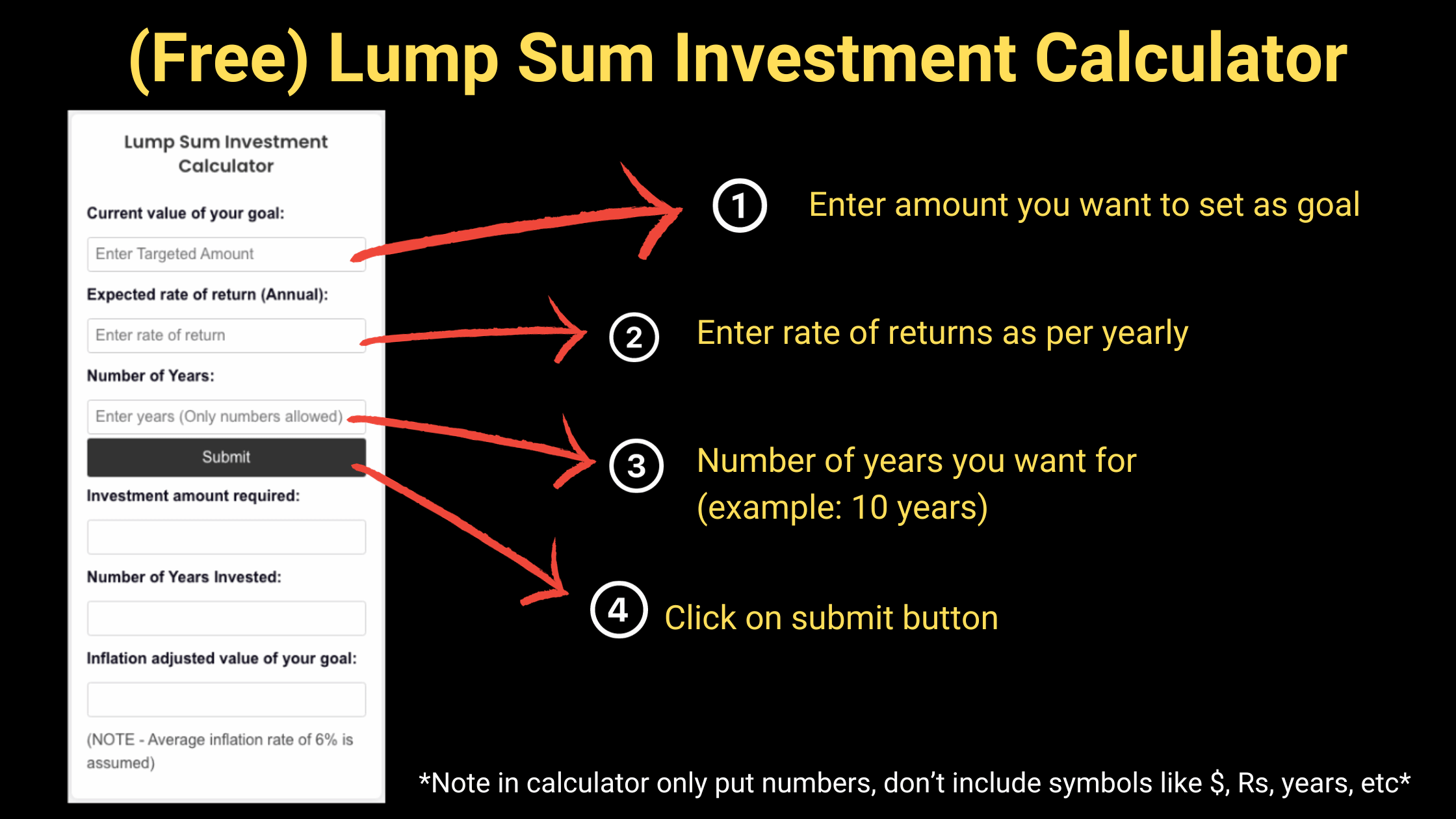lump sum investment calculator

## Lump Sum Investment Calculator: Mutual Fund calculator lumpsum

Lump Sum Investment Calculator:

Lump Sum Investment Calculator

# Lump Sum Investment Calculator

(NOTE - Average inflation rate of 6% is assumed)

Investing is a crucial part of financial planning, and understanding how your investments can grow over time is paramount. Whether you’re a seasoned investor or just starting, the “Lump Sum Investing Calculator” is an invaluable tool that can help you project the future value of your investments.

In this blog, we will guide you through understanding and making the most out of this powerful tool, ensuring you make informed investment decisions.

## Understanding Lump Sum Investment

A lump sum investment is a single, large investment made in a mutual fund or other investment vehicle, as opposed to making smaller, regular investments over time. Many investors prefer this method when they have a significant amount of money available to invest.

## Why Use a Lump Sum Investment Calculator?

With numerous financial tools available, why should you consider using a lump sum investment calculator? Keywords such as “mutual fund calculator lumpsum,” “lumpsum investment calculator,” and “lumpsum mutual fund calculator,” highlight the growing interest in this type of investment strategy.

The “mutual fund lumpsum calculator” offers a straightforward way to estimate the future value of your investment, taking into account variables such as the initial investment amount, the expected annual return rate, and the investment duration.

## Lump Sum Investment Calculator: Your Guide to Smart Investing

### What is this Tool?

The Lump Sum Investment Calculator is a user-friendly tool designed to help investors estimate the future value of their lump sum investment in mutual funds or other investment vehicles.

### How Does it Work?

To provide accurate calculations, the Lump Sum Investing Calculator requires the following inputs:

1. Initial Investment Amount: The amount of money you plan to invest as a lump sum.
2. Expected Annual Return Rate: The annual return rate you anticipate from your investment.
3. Investment Duration: The number of years you plan to stay invested.

The calculator then uses these inputs to compute the future value of your investment, helping you visualize the potential growth of your funds.

### How to Use the Lump Sum Investment Calculator?

Using the Lump Sum Investing Calculator is a breeze. Follow these simple steps:

1. Enter the Initial Investment Amount you plan to invest.
2. Specify the Expected Annual Return Rate for your investment.
3. Choose the Investment Duration in years.
4. Click on the “Calculate” button.
5. View the estimated future value of your investment displayed on the screen.

### Example:

Let’s go through an example to better understand how the calculator works:

• Initial Investment Amount: \$10,000
• Expected Annual Return Rate: 8%
• Investment Duration: 10 years

After entering these values and clicking “Calculate,” the Lump Sum Investing Calculator will display the estimated future value of your investment, helping you gauge the potential growth of your lump sum investment over the specified period.

## Benefits of Using the Lump Sum Investment Calculator

The Lump Sum Investing Calculator is an essential tool for investors looking to maximize their returns. It provides clarity and insight into how your investment can grow over time, enabling you to make informed and strategic investment decisions.

## Conclusion

Investing a lump sum amount can lead to significant financial growth, but it requires careful planning and an understanding of how investments work over time.

The Lump Sum Investing Calculator is a robust tool that empowers investors to project the future value of their investments, helping to demystify the investment process and promote informed decision-making.

Harness the power of this tool today and take a giant leap toward achieving your financial goals. Happy investing!